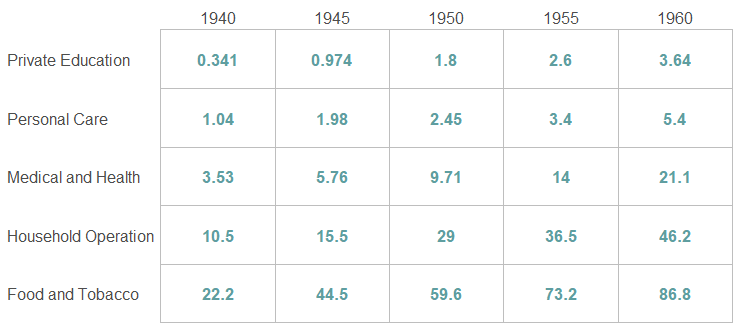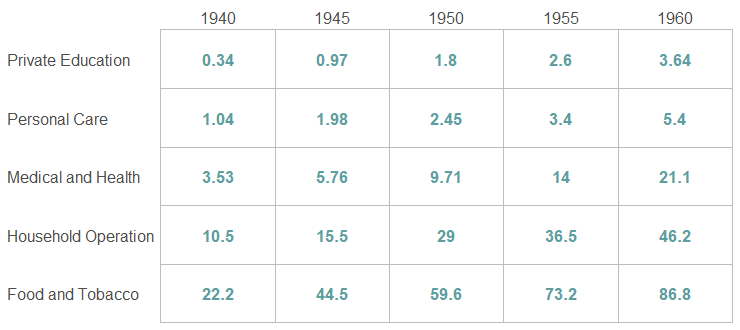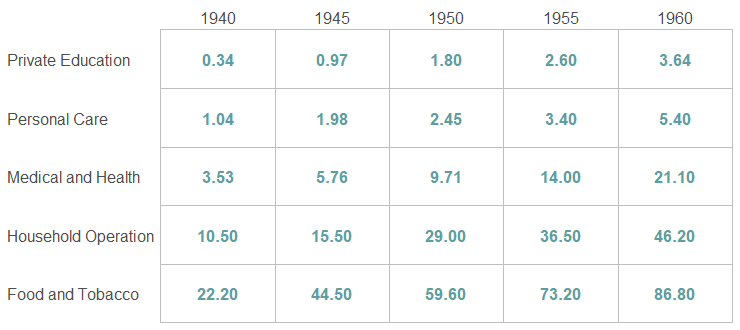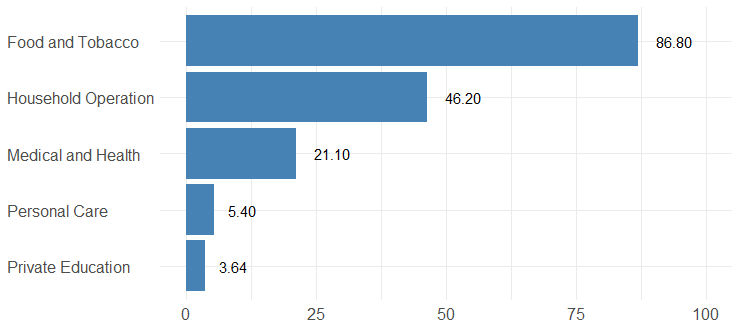# Keep trailing zeros in R ggplot2 geom_text

If you want to keep trailing zeros in R, and in particular for text labels in ggplot2 geom_text, try functions like sprintfformatC, or digits from the formattable package. Add trailing zeros in the R data frame, ggplot2, and keep numerical properties using the function digits from the formattable.

Here is the data set containing United States personal expenditures (in billions of dollars) for a few years. Data about expenditures contain more decimal places than in need.

```library(reshape2)

ue <- melt(USPersonalExpenditure)
ue <- setNames(ue, c("categories", "year", "expenditures"))

#           categories years expenditures
# 1    Food and Tobacco  1940       22.200
# 2 Household Operation  1940       10.500
# 3  Medical and Health  1940        3.530
# 4       Personal Care  1940        1.040
# 5   Private Education  1940        0.341
# 6    Food and Tobacco  1945       44.500```

By using ggplot2, I created a simple table. As you can see, the numbers that I obtained with geom_text have differences in decimal places. I would like to have two everywhere and add trailing zeros where necessary.

```require(ggplot2)

ggplot(ue, aes(x = year, y = categories)) +
geom_tile(colour = "gray", linewidth = 0.25, fill = "white") +
geom_text(aes(label = expenditures)
, color = "cadetblue", size = 4.5, fontface = "bold") +
scale_x_continuous(expand = c(0,0), position = "top") +
scale_y_discrete(expand = c(0,0)) +
theme(axis.title = element_blank()
, text = element_text(size = 15)
, axis.ticks = element_blank()
, axis.text.y = element_text(hjust = 0))```My first idea was to round numbers and keep only two decimal places.

```ue\$expenditures <- round(ue\$expenditures, digits = 2)

#           categories year expenditures
# 1    Food and Tobacco 1940        22.20
# 2 Household Operation 1940        10.50
# 3  Medical and Health 1940         3.53
# 4       Personal Care 1940         1.04
# 5   Private Education 1940         0.34
# 6    Food and Tobacco 1945        44.50```

Unfortunately, in the results, you can see that those who had three decimal places now have two, but those who had one, have only one. There are no trailing zeros where necessary.

```ggplot(ue, aes(x = year, y = categories)) +
geom_tile(colour = "gray", linewidth = 0.25, fill = "white") +
geom_text(aes(label = expenditures)
, color = "cadetblue", size = 4.5, fontface = "bold") +
scale_x_continuous(expand = c(0,0), position = "top") +
scale_y_discrete(expand = c(0,0)) +
theme(axis.title = element_blank()
, text = element_text(size = 15)
, axis.ticks = element_blank()
, axis.text.y = element_text(hjust = 0))```## Keep trailing zeros in R ggplot2geom_text

You can keep trailing zeros in R similarly to adding leading zeros.

Try functions like sprintf or formatC. The result of both functions has character formatting.

```class(sprintf("%.2f", ue\$expenditures))

# "character"```

If you want to get trailing zeros in ggplot2 and keep numerical properties, try the function digits from the formattable.

```class(formattable::digits(ue\$expenditures, digits = 2))

# "formattable" "numeric"

mean(sprintf("%.2f", ue\$expenditures))

# NA

mean(formattable::digits(ue\$expenditures, digits = 2))

# 20.07```

With the function digits, I can overwrite necessary data frame content and keep it shorter with ggpplot2. You can also use that inside geom_text to get trailing zeros where that is necessary.

```ggplot(ue, aes(x = year, y = categories)) +
geom_tile(colour = "gray", linewidth = 0.25, fill = "white") +
geom_text(aes(label = formattable::digits(expenditures, digits = 2))
, color = "cadetblue", size = 4.5, fontface = "bold") +
scale_x_continuous(expand = c(0,0), position = "top") +
scale_y_discrete(expand = c(0,0)) +
theme(axis.title = element_blank()
, text = element_text(size = 15)
, axis.ticks = element_blank()
, axis.text.y = element_text(hjust = 0))```Here is how to get trailing zeros with the sprintf and visualize text labels with two decimal places and trailing zeros.

```ggplot(ue, aes(x = year, y = categories)) +
geom_tile(colour = "gray", linewidth = 0.25, fill = "white") +
geom_text(aes(label = sprintf("%.2f", expenditures))
, color = "cadetblue", size = 4.5, fontface = "bold") +
scale_x_continuous(expand = c(0,0), position = "top") +
scale_y_discrete(expand = c(0,0)) +
theme(axis.title = element_blank()
, text = element_text(size = 15)
, axis.ticks = element_blank()
, axis.text.y = element_text(hjust = 0))```

Here is how to get the same result with the formatC.

```ggplot(ue, aes(x = year, y = categories)) +
geom_tile(colour = "gray", linewidth = 0.25, fill = "white") +
geom_text(aes(label = formatC(expenditures, format = 'f', digits = 2))
, color = "cadetblue", size = 4.5, fontface = "bold") +
scale_x_continuous(expand = c(0,0), position = "top") +
scale_y_discrete(expand = c(0,0)) +
theme(axis.title = element_blank()
, text = element_text(size = 15)
, axis.ticks = element_blank()
, axis.text.y = element_text(hjust = 0))```

The same principle applies to other ggplot2 visualizations where you want to add data labels. Keep a certain amount of decimal places and add trailing zeros where necessary.

Here is how it looks with the bar chart from the previous post about functions sub and gsub.

```require(dplyr)

ue\$expenditures <- formattable::digits(ue\$expenditures, digits = 2)

ue %>%
filter(year == 1960) %>%
ggplot(aes(x = expenditures, y = reorder(categories, expenditures))) +
geom_col(fill = "steelblue") +
geom_text(aes(label = expenditures), hjust = -0.5) +
xlim(0, 100) +
theme_minimal() +
theme(axis.title = element_blank()
, axis.text.y = element_text(hjust = 0)
, text = element_text(size = 15))```Posted

in